Lab 04 MOS Device Characterization

Hung, Bill Chun Wai

Objectives

Find the device parameters for an n-channel MOSFET. From the parameters, you will reproduce its I-V characteristics and compare them to

SPICE. The characteristics will be compared to the SPICE level 1 model. We will also

compare your data with data from the HP 4155 analyzer. The key concepts you should

learn in this lab are:

determining which region of operation the MOSFET is in depending on the values

of VGS and VDS,

application of correct equations for ID depending on the region of operation,

extraction of basic SPICE parameters from experimental measurements

Procedure/Measurement Report

Results/AnalysisFigure 1. Figure 4 Circuit SetupFigure 2. Drain Current Versus Vds Plot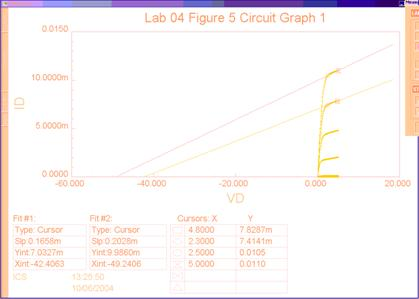Figure 3.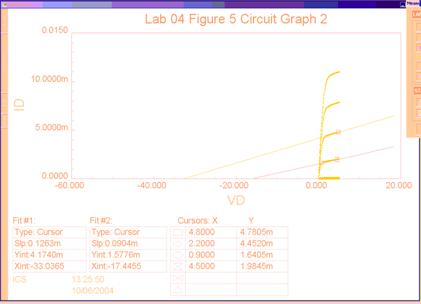Figure 4.

Procedure 3.0 Part 4. Determine Lamda,n from the plot ¡°Lab 04 Figure 4 Circuit¡±

Using, Lamda,n = -1/(x-intercept)

The graph from Figure 2, is rescaled into Figure 3 and Figure 4 above. The tangent lines of the saturation regions are shown. Theoretically, the x-intercepts should be the same, but the experimental values differs. So the average of the data is calculated.

 X-Intercept (V) Lamda, n = -1/X-Intercept (V^-1) -42.4063 0.02358 -49.2406 0.02031 -33.0365 0.03027 -17.4455 0.05732 Average:     0.03287

The average value of Lamda,n  is 0.03287 V^-1, which is also the experimental value of Lamda,n

Procedure 3.0 Part 5

The expected Vd,sat is 2V when Vgs is 3V, and the measured Vd,sat is 1.7V.

The expected Id,sat is 7mA for Vgs is 3V, and the measured Id,sat is 5mA.

The shape of the Figure 2 is the shape of Id vs. Vds curve. Both the expected and the measured curve are slightly upsloping in the saturation region.

Procedure 3.0 Part 6.

The printout is shown in Figure 2 to Figure 4.

Procedure 3.1 Part 2

The equation for Id when the MOSFET is operating in the triode region is

Id = u* Cox (W/L) (Vgs ¨C Vtn ¨C Vds/2) Vds

Procedure 3.1 Part 3 Determination of Vtn and Kn Parameter in the Triode Region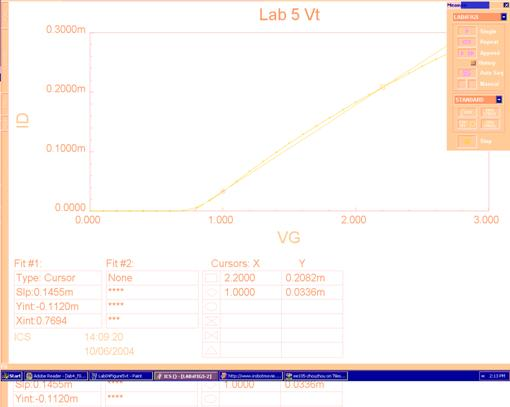Figure 5. Id versus Vgs

Since Id = u* Cox (W/L) (Vgs ¨C Vtn ¨C Vds/2) Vds

Id = u* Cox (W/L)*Vds*Vgs - u* Cox (W/L)*Vds*Vtn - u* Cox/2*(W/L)*Vds^2

So the slope of the graph is

u* Cox (W/L)*Vds = 0.1455 mS (from Figure 5), whereW/L = 46.5/1.5

Kn = u* Cox  = 0.1455mS / ( (46.5/1.5) *50mV) = 93.9 uA/V^2

Vn is the x-intercept of Figure 5.

Vn = 0.8 V

Procedure 3.1 Part 4

Printout is shown in Figure 5

Procedure 3.2 Part 2

The equation for Id when the MOSFET is operating in the triode region is

Id = u* Cox (W/2L) (Vgs ¨C Vtn)^2

Procedure 3.2 Part 3 Determination of Vtn and Kn Parameter in the Saturation Region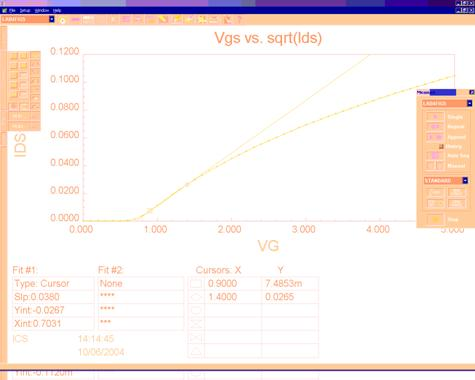Figure 6. sqrt(Id) versus Vgs

Since Id = u* Cox (W/2L) (Vgs ¨C Vtn)^2, where W/L = 46.5/1.5

Sqrt(Id) = sqrt (u* Cox (W/2L) ) (Vgs ¨C Vtn)

Slope = sqrt (u* Cox (W/2L) ) = 0.0380

Kn = u*Cox = 0.0380^2 / (46.5/(1.5*2)) = 93.2 uA/V^2

Vn is the x-intercept of Figure 6.

Vn = 0.8 V

Procedure 3.2 Part 4

Printout is shown in Figure 6

Procedure 3.3 Comparison with PSpice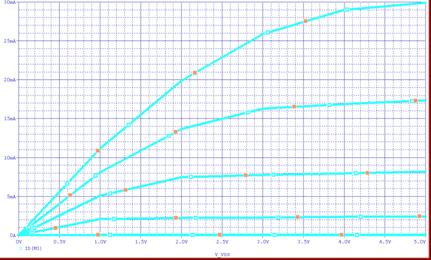Figure 7. Id vs. Vds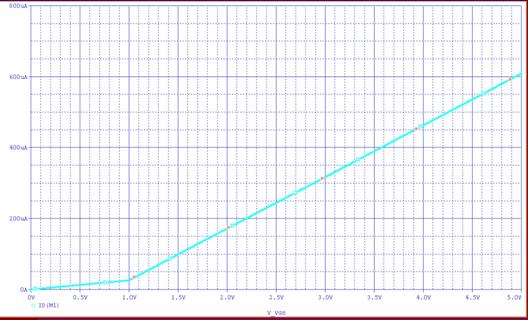Figure 7. Id vs. Vgs

PSpice Stimulation with NMOS model of parameters determined by experiment

.model NMOSFET NMOS(KP=93.9U VTO=0.8 L=1.5U W=46.5U Lambda=0.0328)

PSpice plot of Figure 7 is almost  the same as the experiment plot shown in Figure 1. Both plots have Id,sat of

 Vgs(V) Id(mA) 0 0 1 1 2 2.5 3 8 4 18 5 20

Conclusions

The experimental values determined are

Lamda,n   = 0.03287 V^-1

When the MOSFET is in Triode

Kn = 93.9 uA/V^2

Vn = 0.8 V

When the MOSFET is in Saturation

Kn = 93.2 uA/V^2

Vn = 0.8 V

In this lab, the technique to extract basic parameters are learned. Determination of the state of the MOSFET by observing Vgs and Vds, using correct equations for different the region of operation are learned during the lab.

One of the difficulties about the lab is the graphs are too rough to determine an exact value.